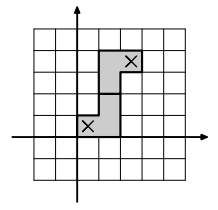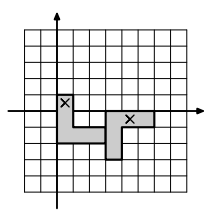시간 제한메모리 제한제출정답맞힌 사람정답 비율
3 초 512 MB47111126.190%

## 문제

Fiora is a game designer. Now she is designing the final level for her new game.

A level for this game is a labyrinth on a rectangular grid with lots of enemies. Player starts her game at the square (0, 0) and her purpose is to get to the square (a, b). Fiora has lots of ideas on how to put enemies, but she does not like designing labyrinths. She needs your help here.

Fiora is drawing levels in a special level editor which supports one basic block to design a labyrinth. This block is an L-shaped corner, consisting of two perpendicular rectangles 1 × n squares in size intersecting at 1 × 1 square. It is possible to rotate this block in four ways. Blocks cannot intersect, but they can touch each other. Player can move through all the squares lying in any block. She can move between two squares if they are sharing a side, even if they are in different blocks.Blocks with n = 3 The first example The second example

Fiora wants to design the final level with the minimal possible number of blocks. Of course, it should be possible to move from square (0, 0) to square (a, b).

## 입력

The first line of the input consists of a single integer m (1 ≤ m ≤ 100) — the number of test cases. It is followed by m test cases. Each test case is on a separate line and consists of three integers a, b, and n (−108 ≤ a, b ≤ 108; 2 ≤ n ≤ 108) — a is the coordinate of the final point along the horizontal axis, b is the coordinate of the final point along the vertical axis, and n is the size of the block. The final point is not same as the starting one (either a ≠ 0 or b ≠ 0).

## 출력

For each test case, in the first line print the minimal number k of blocks you need. In the following k lines print description of these blocks. Each L-shaped corner block is described by coordinates of two cells. Print coordinates of the end of its vertical rectangle, followed by coordinates of the end of its horizontal rectangle. Specify the coordinates of the ends that are opposite to the intersection of the rectangles. Note that the order of cells in the block description matters, since a change of the order results in a reflected block. Coordinates of each end should be printed with the coordinate along the horizontal axis first, followed by the coordinate along the vertical axis.

All coordinates in the output should not exceed 109 by absolute value.

It is guaranteed that the total number of blocks in the correct output does not exceed 105 for all test cases combined.

## 예제 입력 1

2
2 3 2
4 -1 3


## 예제 출력 1

2
1 1 0 0
1 2 2 3
2
0 0 2 -2
3 -3 5 -1